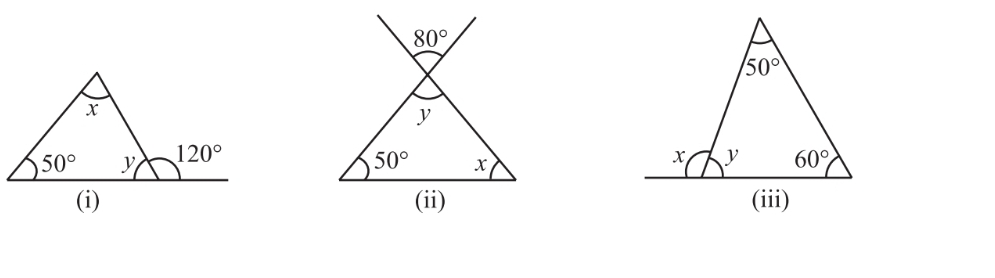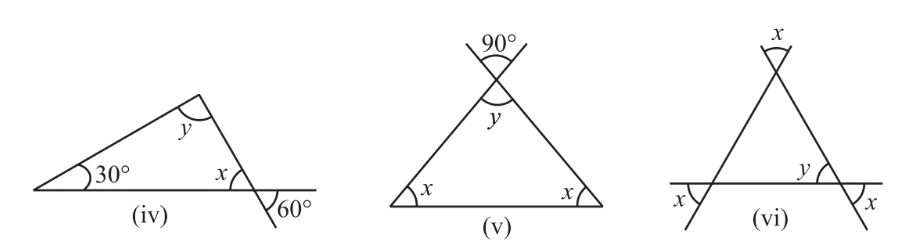# 2. Find the values of the unknowns x and y in the following diagramsi) As we know, the exterior angle is equal to the sum of opposite internal angles in a triangle.Now, As we know the sum of internal angles of a triangle is 180. so,Hence,.

ii)  As we know when two lines are intersecting, the opposite angles are equal. SoNow, As we know the sum of internal angles of a triangle is 180. so,Hence,.

iii) As we know, the exterior angle is equal to the sum of opposite internal angles in a triangleNow, As we know the sum of internal angles of a triangle is 180. so,Hence,.

iv)

As we know when two lines are intersecting, the opposite angles are equal. SoNow, As we know the sum of internal angles of a triangle is 180. so,Hence,v) As we know when two lines are intersecting, the opposite angles are equal. SoNow, As we know the sum of internal angles of a triangle is 180. so,Hence,vi)As we know when two lines are intersecting, the opposite angles are equal. SoNow, As we know the sum of internal angles of a triangle is 180. so,Hence,.

.

## Related Chapters

### Preparation Products

##### Knockout NEET 2024

Personalized AI Tutor and Adaptive Time Table, Self Study Material, Unlimited Mock Tests and Personalized Analysis Reports, 24x7 Doubt Chat Support,.

₹ 40000/-
##### Knockout NEET 2025

Personalized AI Tutor and Adaptive Time Table, Self Study Material, Unlimited Mock Tests and Personalized Analysis Reports, 24x7 Doubt Chat Support,.

₹ 45000/-
##### NEET Foundation + Knockout NEET 2024

Personalized AI Tutor and Adaptive Time Table, Self Study Material, Unlimited Mock Tests and Personalized Analysis Reports, 24x7 Doubt Chat Support,.

₹ 54999/- ₹ 42499/-
##### NEET Foundation + Knockout NEET 2024 (Easy Installment)

Personalized AI Tutor and Adaptive Time Table, Self Study Material, Unlimited Mock Tests and Personalized Analysis Reports, 24x7 Doubt Chat Support,.

₹ 3999/-